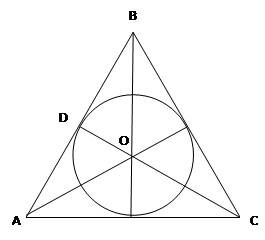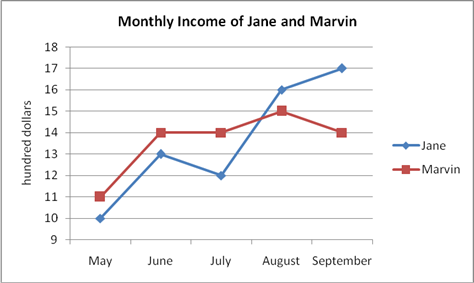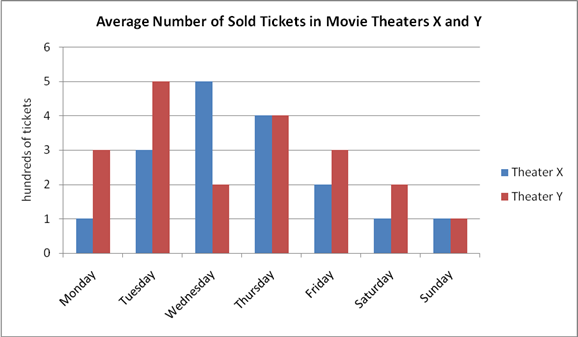### Questions of Test: GRE Quantitative Section practice test

 11 Direction: Each of the Questions 1-15 consists of two quantities, one in Column A and one in Column B. You are to compare the two quantities. Column A Column B ?PQR is a scalene triangle and the measure of angle PQR = 80° The sum of the measure of any two angles of the triangle 100°  12 Direction: Each of the Questions 1-15 consists of two quantities, one in Column A and one in Column B. You are to compare the two quantities. Column A Column B x2 – 2x + 1 = 1 2x x2  13 Direction: Each of the Questions 1-15 consists of two quantities, one in Column A and one in Column B. You are to compare the two quantities. Column A Column BThe circle is inscribed inside an equilateral triangle. The angle bisectors pass through the center of the circle. The measure of angle ADO The measure of angle AOC divided by two  14 Direction: Each of the Questions 1-15 consists of two quantities, one in Column A and one in Column B. You are to compare the two quantities. Column A Column B In 2008, Company A produced 20,000 mobile phones a day, while Company B produces 18,000. In 2009, Company A increased their average daily production by 20% while Company B increased by 30%. The average daily production of Company A in 2009 The average daily production of Company B in 2009  15 Discrete Quantitative Question: -51 - 49 + 47 - 44 + 41 - 37 + 33 16 Discrete Quantitative Question: If 5x – 5 = 20, then x2 = ? 17 Discrete Quantitative Question: Which is closest to  18 Data Interpretation Question: The graph represents the monthly income of Jane and Marvin from May to September. What month is the increase in their combined income greatest?19 Data Interpretation Question:Which of the following is the best estimate of the daily average number of tickets sold in Theater X? 20 Data Interpretation Question:What is the average weekly number of tickets sold in Theater Y?